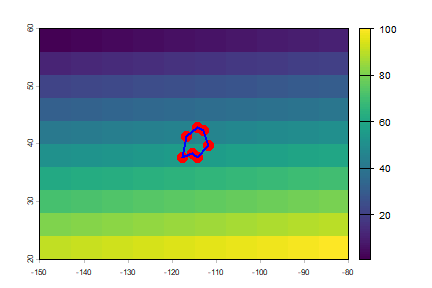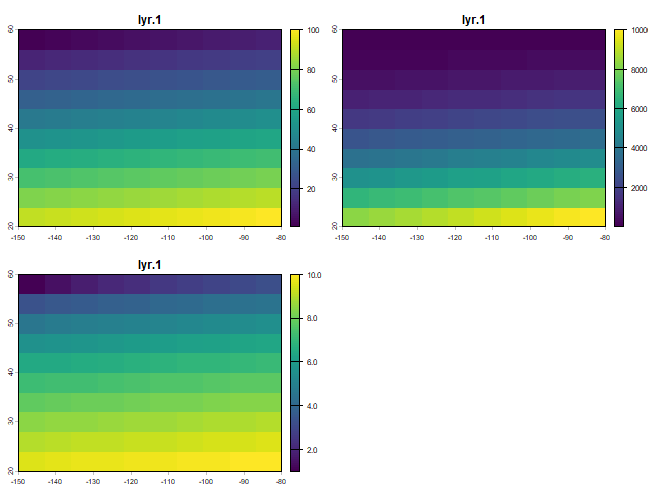# Raster data¶

## Introduction¶

The `terra` package has functions for creating, reading, manipulating, and writing raster data. The package provides, among other things, general raster data manipulation functions that can easily be used to develop more specific functions. For example, there are functions to read a chunk of raster values from a file or to convert cell numbers to coordinates and back. The package also implements raster algebra and many other functions for raster data manipulation.

## SpatRaster¶

A `SpatRaster` represents multi-layer (multi-variable) raster data. A SpatRaster always stores a number of fundamental parameters decribing its geometry. These include the number of columns and rows, the spatial extent, and the Coordinate Reference System. In addition, a `SpatRaster` can store information about the file in which the raster cell values are stored. Or, if there is no such a file, a `SpatRaster` can hold the cell values in memory.

Here I create a `SpatRaster` from scratch. But note that in most cases where real data is analyzed, these objects are created from a file.

```library(terra)
## terra 1.7.23
r <- rast(ncol=10, nrow=10, xmin=-150, xmax=-80, ymin=20, ymax=60)
r
## class       : SpatRaster
## dimensions  : 10, 10, 1  (nrow, ncol, nlyr)
## resolution  : 7, 4  (x, y)
## extent      : -150, -80, 20, 60  (xmin, xmax, ymin, ymax)
## coord. ref. : lon/lat WGS 84
```

SpatRaster `r` only has the geometry of a raster data set. That is, it knows about its location, resolution, etc., but there are no values associated with it. Let’s assign some values. In this case I assign a vector of random numbers with a length that is equal to the number of raster cells.

```values(r) <- runif(ncell(r))
r
## class       : SpatRaster
## dimensions  : 10, 10, 1  (nrow, ncol, nlyr)
## resolution  : 7, 4  (x, y)
## extent      : -150, -80, 20, 60  (xmin, xmax, ymin, ymax)
## coord. ref. : lon/lat WGS 84
## source(s)   : memory
## name        :      lyr.1
## min value   : 0.02905809
## max value   : 0.98547879
```

You could also assign cell numbers (in this case overwriting the previous values)

```values(r) <- 1:ncell(r)
r
## class       : SpatRaster
## dimensions  : 10, 10, 1  (nrow, ncol, nlyr)
## resolution  : 7, 4  (x, y)
## extent      : -150, -80, 20, 60  (xmin, xmax, ymin, ymax)
## coord. ref. : lon/lat WGS 84
## source(s)   : memory
## name        : lyr.1
## min value   :     1
## max value   :   100
```

We can plot this object.

```plot(r)
lon <- c(-116.8, -114.2, -112.9, -111.9, -114.2, -115.4, -117.7)
lat <- c(41.3, 42.9, 42.4, 39.8, 37.6, 38.3, 37.6)
lonlat <- cbind(id=1, part=1, lon, lat)
pts <- vect(lonlat)
pols <- vect(lonlat, type="polygons", crs="+proj=longlat +datum=WGS84")
points(pts, col="red", pch=20, cex=3)
lines(pols, col="blue", lwd=2)
```You can create a multi-layer object using the `c` method.

```r2 <- r * r
r3  <- sqrt(r)
s <- c(r, r2, r3)
s
## class       : SpatRaster
## dimensions  : 10, 10, 3  (nrow, ncol, nlyr)
## resolution  : 7, 4  (x, y)
## extent      : -150, -80, 20, 60  (xmin, xmax, ymin, ymax)
## coord. ref. : lon/lat WGS 84
## source(s)   : memory
## names       : lyr.1, lyr.1, lyr.1
## min values  :     1,     1,     1
## max values  :   100, 10000,    10
plot(s)
```